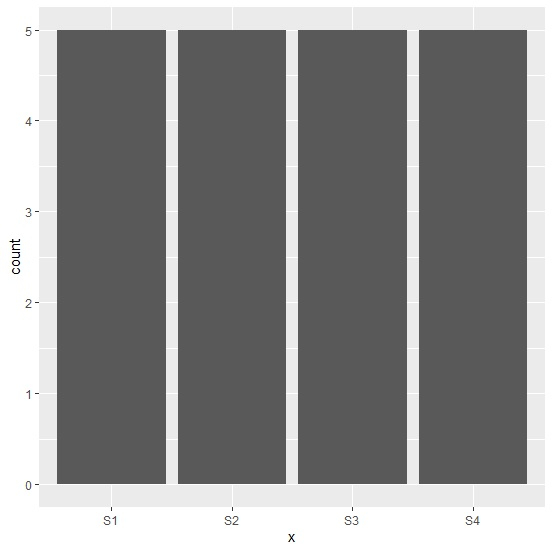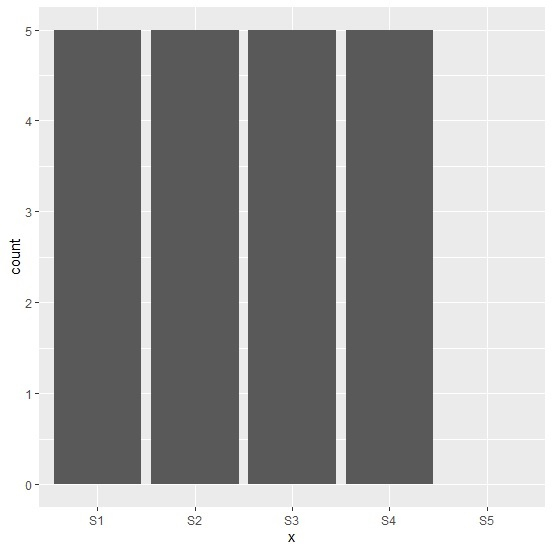# How to include a factor level in bar blot using ggplot2 in R if that level has a frequency zero.

In research, sometimes we get a count of zero for a particular level of a factor variable but we might want to plot that in the bar plot so that anyone who look at the plot can easily understand what is missing and compare all the factor levels. In ggplot2, it can be done with the help of scale_x_discrete function.

> x<-factor(rep(c("S1","S2","S3","S4"),times=5))
> df<-data.frame(x)

Suppose you have a factor level S5 as well but the frequency for S5 is zero as shown below −

> df$x<-factor(df$x,levels=c("S1","S2","S3","S4","S5"))
> df\$x
 S1 S2 S3 S4 S1 S2 S3 S4 S1 S2 S3 S4 S1 S2 S3 S4 S1 S2 S3 S4
Levels: S1 S2 S3 S4 S5

> library(ggplot2)

Now when we plot the bar plot the fifth level is not there −

> ggplot(df,aes(x))+geom_bar()

## OutputThe R command to plot all the factor levels is as shown below −

> ggplot(df,aes(x))+geom_bar()+
+ scale_x_discrete(drop=FALSE)

## Output